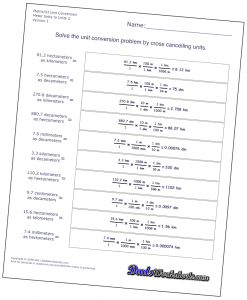# Metric Unit Conversion Worksheets Now AvailableWe're moving up the grades, and the line between math and science classes is starting to blur. For my oldest daughter, this is the first year of chemistry. All of these years I've been saying, "You're going to use math a lot in science class," and I'm finally starting to look smart in a young girl's eyes. So say hello, friends, to unity fractions and the metric conversion worksheets! We typically learn conversions for jumping easily between common units, especially in the metric system where most conversions are just a matter of multiplying or dividing by some power of ten. However, these simple conversions start to break down where the conversions become more complex. Quick, can you convert 64 decaliters into centiliters? No? Yeah, well me either. The way around this is unity fractions. Each unity fraction expresses a conversion from base units (think meters, liters or grams for starters) to derived units (milli units, centi units, kilo units, etc.). By stringing more than one unity fraction together, you can easily convert from one derived unit to another. Units and factors of ten cross-cancel, just like with fraction multiplication, and the process can be extended easily to cover square or cubic units. To get things started, I've posted conversion worksheets for distance (meters), mass (grams) and volume (liters), but you can look forward to more worksheets soon that cover conversions between different classes of units as well as imperial measurements. For now, you can find the new worksheets at the links here... Metric Length Conversion WorksheetsMetric Mass Conversion WorksheetsMetric Volume Conversion Worksheets As always, if you find these worksheets or any others useful, please consider clicking the Google "+1" button near the top of the page to let others know. Your support of the site is much appreciated!